# Excel VLOOKUP not working - solving #N/A and #VALUE errors

Is your VLOOKUP pulling wrong data or you cannot get it to work at all? This tutorial shows how you can quickly fix common VLOOKUP errors and overcome its main limitations.

In a few earlier articles, we explored different aspects of the Excel VLOOKUP function. If you have been following us closely, by now you should be an expert in this area :)

However, it's not without reason that many Excel specialists consider VLOOKUP to be one of the most intricate Excel functions. It has a ton of limitations, which are the source of various problems and errors.In this article, you will find simple explanations of the main causes of VLOOKUP errors such as #N/A, #NAME and #VALUE, as well as their solutions and fixes. We will start with the most obvious reasons why VLOOKUP is not working, so it might be a good idea to check out the below troubleshooting steps in order.

## Fixing #N/A error in VLOOKUP

In VLOOKUP formulas, the #N/A error message (meaning "not available") is displayed when Excel cannot find a lookup value. There can be several reasons why that may happen.

#### 1. The lookup value is misspelt

It's always a good idea to check the most obvious thing first : ) Misprints frequently occur when you are working with really large data sets consisting of thousands of rows, or when a lookup value is typed directly in the formula.

#### 2. #N/A in approximate match VLOOKUP

If your formula looks up the closest match, (range_lookup argument set to TRUE or omitted), the #N/A error can appear in two cases:

• The lookup value is smaller than the smallest value in the lookup array.
• The lookup column is not sorted in ascending order.

#### 3. #N/A in exact match VLOOKUP

If you are searching for exact match (range_lookup argument set to FALSE), the #N/A error occurs when a value exactly equal to the lookup value is not found. For more information, see VLOOKUP exact match vs. approximate match.

#### 4. The lookup column is not the leftmost column of the table array

One of the most significant limitations of Excel VLOOKUP is that it cannot look to its left. Consequently, a lookup column should always be the leftmost column in the table array. In practice, we often forget about this and end up with #N/A errors.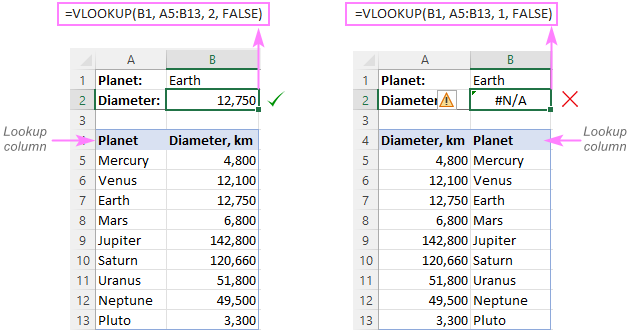Solution: If it is not possible to restructure your data so that the lookup column is the left-most column, you can use the INDEX and MATCH functions together as an alternative to VLOOKUP. Here's a formula example: INDEX MATCH formula to look up values to left.

#### 5. Numbers are formatted as text

Another common source #N/A errors in VLOOKUP formulas is numbers formatted as text, either in the main or lookup table.

This usually occurs when you import data from some external database or if you've typed an apostrophe before a number to show leading zeros.

Here are the most obvious indicators of numbers formatted as text: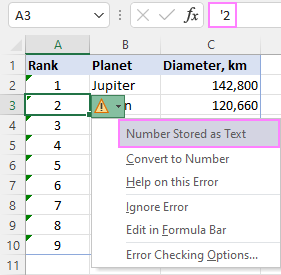Solution: Select all the problematic numbers, click on the error icon and choose Convert to Number from the context menu. For more information, please see How to convert text to number in Excel.

#### 6. Leading or trailing spaces

This is the least obvious cause of the VLOOKUP #N/A error because a human eye can hardly spot those extra spaces, especially when working with big datasets where most of the entries are below the scroll.

Solution 1: Extra spaces in the lookup value

To ensure the correct work of your VLOOKUP formula, wrap the lookup value in the TRIM function:

`=VLOOKUP(TRIM(E1), A2:C10, 2, FALSE)`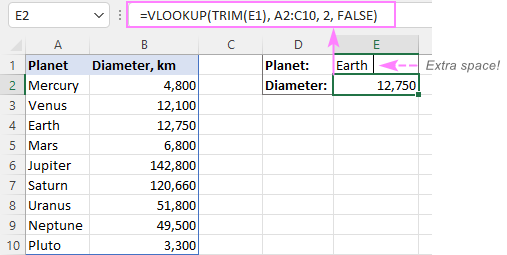Solution 2: Extra spaces in the lookup column

If extra spaces occur in the lookup column, there is no easy way to avoid #N/A errors in VLOOKUP. Instead, you can use a combination of INDEX, MATCH and TRIM functions as an array formula:

`=INDEX(B2:B10, MATCH(TRUE, TRIM(A\$2:A\$10)=TRIM(E1), 0))`

Since this is an array formula, don't forget to press Ctrl + Shift + Enter to properly complete it (in Excel 365 and Excel 2021 where arrays are native, this also works as a regular formula).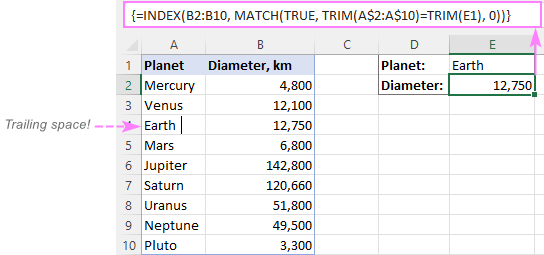Tip. A quick alternative is running the Trim Spaces tool that will eliminate excess spaces both in the lookup and main tables in seconds, making your VLOOKUP formulas error-free.

## #VALUE! error in VLOOKUP formulas

In general, Microsoft Excel displays the #VALUE! error if a value used in the formula is of a wrong data type. In respect to VLOOKUP, there are three common sources of the VALUE! error.

#### 1. Lookup value exceeds 255 characters

Please be aware that VLOOKUP cannot look up values containing more than 255 characters. If your lookup values exceed this limit, a #VALUE! error will be displayed: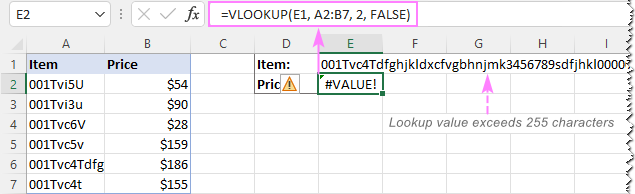Solution: Use an INDEX MATCH formula instead. In our case, this formula works perfectly:

`=INDEX(B2:B7, MATCH(TRUE, INDEX(A2:A7= E1, 0), 0))`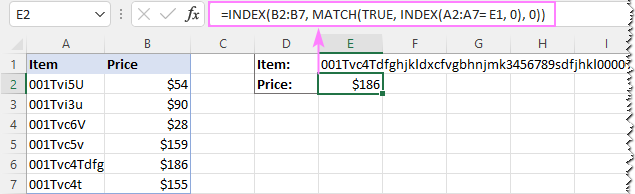#### 2. Full path to the lookup workbook is not supplied

If you are pulling data from another workbook, you have to include the full path to it. More precisely, you have to enclose the workbook's name including the extension in [square brackets] and specify the sheet's name followed by the exclamation mark. If the workbook name or sheet name, or both, contain spaces or any non-alphabetical characters, the path must be enclosed in single quotation marks.

Here's the structure of the table_array argument to Vlookup from another workbook:

`'[workbook name]sheet name'!range`

A real formula might look similar to this:

`=VLOOKUP(\$A\$2,'[New Prices.xls]Sheet1'!\$B:\$D, 3, FALSE)`

The above formula will search for the value of A2 in column B of Sheet1 in the New Prices workbook, and return a matching value from column D.

If any element of the path is missing, your VLOOKUP formula won't work and return the #VALUE error (unless the lookup workbook is currently open).

#### 3. The col_index_num argument is less than 1

It's hard to imagine a situation when someone intentionally enter a number less than 1 to specify the column to return values from. But it may happen if this argument is returned by some other function nested in your VLOOKUP formula.

So, if the col_index_num argument is than 1, your formula will return the #VALUE! error too.

If col_index_num is greater than the number of the columns in the table array, VLOOKUP produces the #REF! error.

## Solving VLOOKUP #NAME error

This is the easiest case - the #NAME? error appears if you've accidentally misspelled the function's name.

The solution is obvious - check the spelling :)

## The main causes of errors in Excel VLOOKUP

Apart from having a fairly complicated syntax, VLOOKUP has arguably more limitations than any other Excel function. Because of these limitations, a seemingly correct formula may often deliver results different from what you expected. Below you will find solutions for a few typical scenarios when VLOOKUP fails.

### VLOOKUP is case-insensitive

The VLOOKUP function does not distinguish the letter case and teats lowercase and uppercase characters as identical.

Solution: Use VLOOKUP, XLOOKUP or INDEX MATCH in combination with the EXACT function that can match text case. You can find the detailed explanations and formula examples in this tutorial: 5 ways to do a case-sensitive Vlookup in Excel.

### A new column was inserted or removed from the table

Regrettably, VLOOKUP formulas stop working every time when a new column is deleted from or added to the lookup table. This happens because the syntax of the VLOOKUP function requires defining the index number of the return column. When a new column is added to/removed from the table array, obviously that index number changes.

Solution: The INDEX MATCH formula comes to the rescue again : ) With INDEX MATCH, you specify the lookup and return ranges separately, so you are free to delete or insert as many columns as you want without worrying about updating every associated formula.

### Cell references change when copying the formula to other cells

The heading gives an exhaustive explanation of the problem, right?

Solution: Always use absolute references (with the \$ sign) for the table_array argument, e.g. \$A\$2:\$C\$100 or \$A:\$C. You can quickly switch between different reference types by pressing the F4 key.

### VLOOKUP returns the first found value

As you already know, Excel VLOOKUP returns the first value it finds. However, you can force it to bring the 2nd, 3rd, 4th or any other occurrence you want. There is also a way to get the last match or all found matches.

Solutions: Formula examples are available here:

## Why does my VLOOKUP work for some cells but not others?

When your VLOOKUP formula returns the correct data I some cells and #N/A errors in others, there can be a few possible reasons why that happens.

1. The table array is not locked

Suppose you have this formula in row 2 (say in E2), which works nicely:

`=VLOOKUP(D2, A2:B10, 2, FALSE)`

When copied to row 3, the formula changes to:

`=VLOOKUP(D3, A3:B11, 2, FALSE)`

Because a relative reference is used for table_array, it changes based on the relative position of the row where the formula is copied, in our case from A2:B10 to A3:B11. So, if the match is in row 2, it won't be found!

Solution: When using a VLOOKUP formula for more than one cell, always lock the table array reference with the \$ sign like \$A\$2:\$B\$10.

2. Text values or data types do not match

Another common reason for VLOOKUP failure is the difference between your lookup value and a similar value in the lookup column. In some cases, the difference is so subtle that it's hard to spot visually.

Solution: When VLOOKUP is returning an #N/A error while you can clearly see the lookup value in the lookup column, and apparently both are spelt exactly the same, the first thing you need to do is to determine the root cause of the problem - the formula or the source data.

To see whether the two values are the same or different, make a direct comparison in this way:

`=E1=A4`

Where E1 is your lookup value and A4 is an identical value in the lookup column.

If the formula returns FALSE, that means the values differ in some way, though they look absolutely alike.

In case of numeric values, the most possible reason is numbers formatted as text.

In case of text values, most likely the problem is in excess spaces. To verify this, find out the total length of the two strings using the LEN function:

`=LEN(E1)`

`=LEN(A4)`

If the resulting numbers are different (like in the screenshot below), then you've pinpointed the culprit - extra spaces: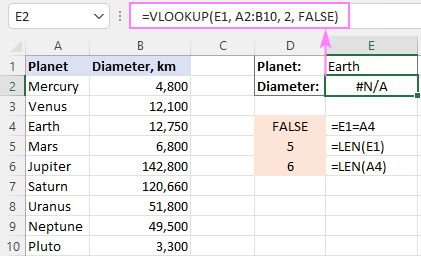To solve the issue, either remove extra spaces or use this INDEX MATCH TRIM formula as a workaround.

## Why does my VLOOKUP pull wrong data?

There could be even more reasons why your VLOOKUP returns a wrong value:

1. Incorrect search mode. If you want an exact match, be sure to set the range_lookup argument to FALSE. The default is TRUE, so if you omit this argument, VLOOKUP will assume you are looking for an approximate match and search for the closest value that is smaller than the lookup value.
2. The lookup column is not sorted. For approximate match VLOOKUP (range_lookup set to TRUE) to work correctly, the first column in the table array must be sorted in ascending order, from smallest to largest.
3. Duplicates in the lookup column. If the lookup column contains two or more duplicate values, VLOOKUP will return the first found match, which may not be the one you expect.
4. Incorrect return column. Double-check the index number in the 3rd argument :)

## VLOOKUP not working between two sheets

First off, it should be noted that the common reasons of #N/A, #VALUE, and #REF errors discussed above may cause the same problems when looking up from another sheet. If it's not the case, check out the following points:

1. Make sure the external reference to another sheet or a different workbook is correct.
2. When doing a Vlookup from another workbook which is closed at the moment, verify that your formula contains the full path to the closed workbook.
3. If VLOOKUP cannot pick a table array in another worksheet (i.e. when you highlight a range in the lookup sheet, nothing appears in the table_array argument in the formula or in the corresponding box of the formula wizard), then most likely the two sheets are open in separate instances of Excel and cannot communicate with each other. For more info, please see How to determine which Excel files are in which instance. To fix this, simply close all Excel windows, and then reopen the sheets/workbooks in the same instance (the default behavior).

## How to Vlookup without errors in Excel

If you do not want to intimidate your users with standard Excel error notations, you can display your own user-friendly text instead or return a blank cell if nothing is found. This can be done by using VLOOKUP with IFERROR or IFNA function.

### Catch all errors

In Excel 2007 and later, you can use the IFERROR function to check a VLOOKUP formula for errors and return your own text (or an empty string) if any error is detected.

For example:

`=IFERROR(VLOOKUP(E1, A2:B10, 2, FALSE), "Oops, something went wrong")`

In Excel 2003 and earlier, you can use the IF ISERROR formula for the same purpose:

`=IF(ISERROR(VLOOKUP(E1, A2:B10, 2, FALSE)), "Oops, something went wrong", VLOOKUP(E1, A2:B10, 2, FALSE))`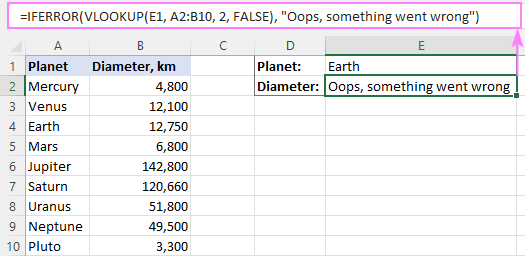For more details, please see Using IFERROR with VLOOKUP in Excel.

### Handle #N/A errors

To trap only #N/A errors ignoring all other error types, use the IFNA function (in Excel 2013 and higher) or IF ISNA formula (in all versions).

For example:

`=IFNA(VLOOKUP(E1, A2:B10, 2, FALSE), "Oops, no match is found. Please try again!")`

`=IF(ISNA(VLOOKUP(E1, A2:B10, 2, FALSE)), "Oops, no match is found. Please try again!", VLOOKUP(E1, A2:B10, 2, FALSE))`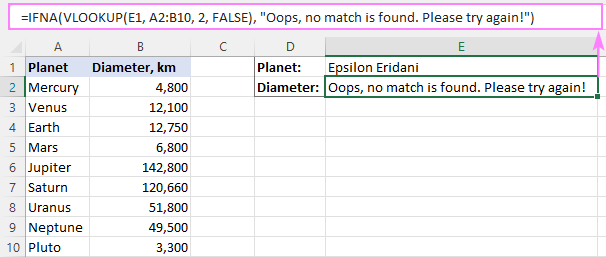That's all for today. Hopefully, this tutorial will help you get rid of VLOOKUP errors and have your formulas working in the way you want.

## You may also be interested in

1. I have issues with my VLOOKUP as my table range data has exceeded and I did tried to change the range still it is giving me #N/A any solution is really appreciated
My Initial Formula
=VLOOKUP(ROW()-8,\$C\$9:\$E\$500,2,FALSE) where
C\$9 value is 482
Row value is 502
Where as E\$500 is =IF(register!C495="",register!A495,0)
and it was meant to return the value whenever E is filled
and C column also contain formula =COUNTIF(G\$9:G\$700,H502)+1
=COUNTIF(G\$9:G\$700,H502)+1 =ROW() =IF(register!C497="",register!A497,0)
=IF(E502=0,CONCATENATE("zzzz",ROW()),IF(register!M497=0,"",CONCATENATE(register!M497,E502,ROW()))) =CONCATENATE("<",G502)
=VLOOKUP(ROW()-8,\$C\$9:\$E\$700,2,FALSE)

I tried by changing range to 700 on each one still not able to solve the problem with N/A

• Hi! I can't check your formula because I don't have your data. If you have a specific question about a function or formula, feel free to ask.

2. I am using the vlookup formula, and i can not password protect the sheets as my whole department needs to access them. I use the vlookup successfully and Crtl+C and ctrl+v isn’t pasting the values from the other sheet without the formula and giving a locked formula error so all of the changes are affecting the original sheet as well.

• Paste with CTRL+SHIFT+V (Paste As Special.. 'Value').

3. I am using a Vlookup that pulls in dates. It is pulling in the dates with the format of 7/15/2311 instead of 7/15/2022. I am not sure how to fix it as I have tried and can't get it to work properly. This is probably and easy fix and I'm just missing something simple, but I need some assistance please.

4. Hi ,

I am using VLookup to compare data between two columns present in different sheet of same workbook. It works fine for all but if the column has empty values then it throws NA .Ex; C2 in first sheet is empty and c2 in second sheet is empty as well

• Hi!
VLOOKUP cannot search for an empty value. Use the recommendations from the article above.

5. Greetings,

My VLOOKUP, will not give me the data I need. I need data from column 13. It will give the correct data from every other column except 13. What am I doing wrong? Please help.

• Hi!
I can't help as I can't see your data and your formula.

6. Hi everyone,
If you are reading this post then you have an issue with VLookup. I spent hours trying to work out the problem.
For me the problem when copying down / pasting the VLookup formula as that my calculations were set to manual.
Go into excel and in the search bar type "Calculation" and see if Automatic is selected or if for some reason Manual is selected.
Change to Automatic and hopefully your VLoopup past will now work.

• omg thank you.

7. thank you, it was helpful

8. I have a VLOOKUP issue that I don't understand. Any thoughts?

This VLOOKUP works as expected and gives me what I want

=VLOOKUP([@[TheName]],Table2[FixedName],1,FALSE)

That tells me that all of the entries in my TheName list are also in my FixedName list in Table2.

My problem is that this next VLOOKUP does not work. Table2 has 10 columns, and 2 is FixedName and column 3 is TheRole. I want to lookup by FixedName as before, but I want the second column, TheRole, returned.

=VLOOKUP([@[TheName]],Table2[@[FixedName]:[TheRole]],2,FALSE)

This works for some rows, but for other rows I get #N/A.

• Hi!
I can't check the formula that contains unique references to your workbook worksheets, sorry. If the formula works for some values, then the problem is not in the formula, but in the values.